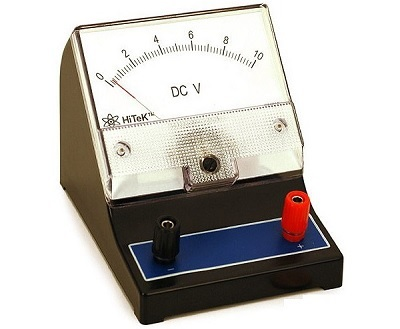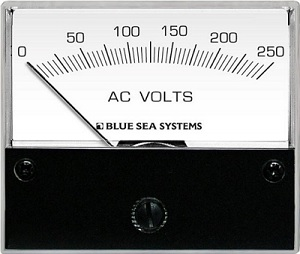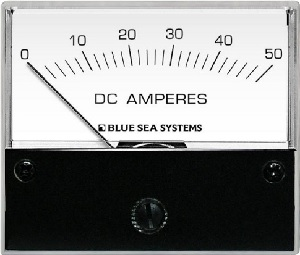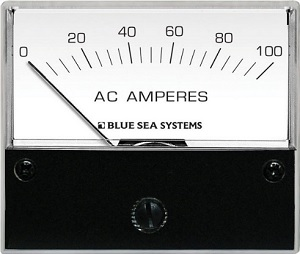# Electronic Measuring Instruments

The instruments used to measure any quantity are known as measuring instruments. If the instruments can measure the basic electrical quantities, such as voltage and current are known as basic measuring instruments.

## Types of Basic Measuring Instruments

We can classify the basic measuring instruments into the following two types.

• Voltmeters
• Ammeters

Let us discuss about these two basic measuring instruments briefly.

### Voltmeters

As the name suggests, voltmeter is a measuring instrument which measures the voltage across any two points of an electric circuit. The units of voltage are volt and the measuring instrument is meter. Hence, the word “voltmeter” is obtained by combining the two words “volt” and “meter”.

We can classify the voltmeters into the following two types based on the type of voltage that it can measure.

• DC Voltmeters
• AC Voltmeters

### DC Voltmeter

As the name suggests, DC voltmeter measures the DC voltage across any two points of an electric circuit. A practical DC voltmeter is shown in below figure.The DC voltmeter shown in the figure is a $(0-10)V$ DC voltmeter. Hence, it can be used to measure the DC voltages from zero volts to 10 volts

### AC Voltmeter

As the name suggests, AC voltmeter measures the AC voltage across any two points of an electric circuit. A practical AC voltmeter is shown in below figure.The AC voltmeter shown in above figure is a $(0-250)V$ AC voltmeter. Hence, it can be used to measure the AC voltages from zero volts to 250 volts

### Ammeters

As the name suggests, ammeter is a measuring instrument which measures the current flowing through any two points of an electric circuit. The unit of current is ampere and the measuring instrument is meter. The word “ammeter” is obtained by combining “am” of ampere with “meter”.

We can classify the ammeters into the following two types based on the type of current that it can measure.

• DC Ammeters
• AC Ammeters

### DC Ammeter

As the name suggests, DC ammeter measures the DC current that flows through any two points of an electric circuit. A practical DC ammeter is shown in figure.The DC ammeter shown in above figure is a $(0-50)A$ DC ammeter. Hence, it can be used to measure the DC currents from zero Amperes to 50 Amperes

### AC Ammeter

As the name suggests, AC ammeter measures the AC current that flows through any two points of an electric circuit. A practical AC ammeter is shown in below figure.The AC ammeter shown in above figure is a $(0-100)A$ AC ammeter. Hence, it can be used to measure the AC currents from zero Amperes to 100 Amperes.

We will discuss about various voltmeters and ammeters in detail in the following few chapters